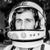## Julia Community 🟣# Creating a simple function for factorial in Julia

Introduction

This article aims to show how you can create a simple function in Julia to get the factorial of a given number.

What is factorial?

The product of all integers equal to or less in value than the original number — in other words, a factorial is the number of possible combinations with numbers less than or equal to that number.

We symbolise the factorial of a number with “!” (e.g. 4!).

Some examples

2!=2∙1=2

3!=3∙2∙1=6

4!=4∙3∙2∙1=24

Logical zero factorial proof

As we said, a factorial is the number of possible combinations with numbers less than or equal to that number. Zero has no numbers less than it, but it is a number itself. How many arrangements can I do with 0 items? None at all. This is too a way of arrangement, so 0!=1 (none).

Suppose we have three fruits:

🍎🍐🍊

What are the arrangements?

🍎🍐🍊
🍎🍊🍐
🍐🍎🍊
🍐🍊🍎
🍊🍎🍐
🍊🍐🍎
3!=6 as we said previously.

Think similarly for 0!.

Function in Julia

``````function f(n::Int64)
f = 1
if n < 0
print("Factorial does not exist for negative numbers")
end
if n == 0
print("The factorial of 0 is 1")
end
if n>0
for i in 1:n
f = f*i
end
println("The factorial is: ",f)
end
end
``````

Let’s ask the factorial of -3 (n=-3)
`f(-3)`

Output:

Factorial does not exist for negative numbers

Let’s ask the factorial of 0 (n=0)
`f(0)`

Output:

The factorial of 0 is 1

Let’s ask the factorial of 3 (n=3)
`f(3)`

Output:

The factorial is: 6

Let’s explain the part of the code below:

``````for i in 1:n
f = f*i
end
``````

Firstly, we defined the f as 1.

for i in 1:3

1. f=1*1=1
2. f=1*2=2
3. f=2*3=6 end

That’s simple!

Conclusion

In this article, we gave a simple explanation of the factorial as well we made an easy function in Julia!

Article Cover Photo: Photo by Tommy Bond on UnsplashMiguel Raz Guzmán Macedo

I liked this article - I wrote a similar one when I was starting out with Julia too!
And then a kind Teacher Assistant for my course came along (with a sneaky smile) and whispered something about some ternary operator...

``````myfact(n::Int) = n == 0 ? 1 : n*myfact(n-1)
``````

...Which creeped me out.

Vexed me!

But not as much as when I found out about the world of map, reduce, and folds, and all those nifty iterator methods that can get you out of a pinch:

``````myfact(n::Int) = prod(big(1):n)
``````